# Hillshade, colors and marginal plots with tidyterra (II)

The rain in Spain does not stay mainly in the plain

This is the second post of the series “Hillshade, colors and marginal plots with tidyterra”. In this post I would explore an approach for annotating marginal plots to a ggplot2 map of a SpatRaster, including information of the values by longitude and latitude. See the first post of the series here.

If you love watching classic movies, specially from the Hollywood’s Golden Age, you may recognize the following lyrics:

The rain in Spain stays mainly in the plain!

By George, she’s got it! By George, she’s got it!

Now, once again where does it rain? On the plain! On the plain!

And where’s that soggy plain? In Spain! In Spain!

The rain in Spain stays mainly in the plain!

The rain in Spain stays mainly in the plain!

This hard statement is made on My Fair Lady (1964) by Audrey Hepburn, Rex Harrison and Stanley Holloway. But as a Spaniard I can tell it is completely false.

The rain in Spain stays mainly in the north, most notably in Galicia. And I can prove it!

On this post I would overlay a SpatRaster showing average precipitation data with an extra set of plots on the margin to identify where the rain in Spain stays (mainly).

## Libraries

On this post we would use the following libraries:

``````## Libraries

# Data manipulation
library(terra)
library(tidyterra)
library(dplyr)

# Get the data
library(geodata)
library(mapSpain)

# Plotting
library(ggplot2)
library(scales)
library(cowplot)
library(colorspace)
``````

## The plain in Spain

Well, the plain (or as we name it La Meseta Central) covers a large area of the inner land of Spain, with an average altitude of 650 meters over the sea level.

I didn’t find any accurate spatial data file with the bounds of the plain, so for this case I would approximate it using a mixture of political borders (historically the Meseta is associated to Castile and Madrid) and elevation data to get a rough shape.

``````
# Using mapSpain
the_plain <- esp_get_prov(
c(
"Teruel"
),
epsg = 4326, resolution = 1
) %>%
mutate(the_plain = TRUE) %>%
group_by(the_plain) %>%
# Combine
summarise() %>%
# To terra, until this step was sf
vect()

# Get altitude

# I use here a local directory to cache downloaded files on my PC.
# Modify this to your likes, e.g. using
# mydir <- tempdir()

mydir <- "~/R/mapslib/misc"

r_init <- elevation_30s("ESP", path = mydir)

# For better handling we set here the names
names(r_init) <- "alt"

# We don't want values lower than 0 on the raster
r <- r_init %>%
mutate(alt = pmax(0, alt))

# Now intersect the raster and the vector and filter by range

exploded <- r %>%
# Let's define here a range of elevations
filter(alt > 600 & alt < 1100) %>%
drop_na() %>%
as.polygons(dissolve = TRUE, na.rm = TRUE) %>%
# Aggregate first
aggregate() %>%
# Explode vectors
disagg() %>%
# And fill holes
fillHoles()

# Select biggest polygons (area bigger than 50 kms 2)
r_plain <- exploded %>%
mutate(area = expanse(exploded)) %>%
filter(area > 50000**2) %>%
# And convert to lines
as.lines()

autoplot(r_plain)
``````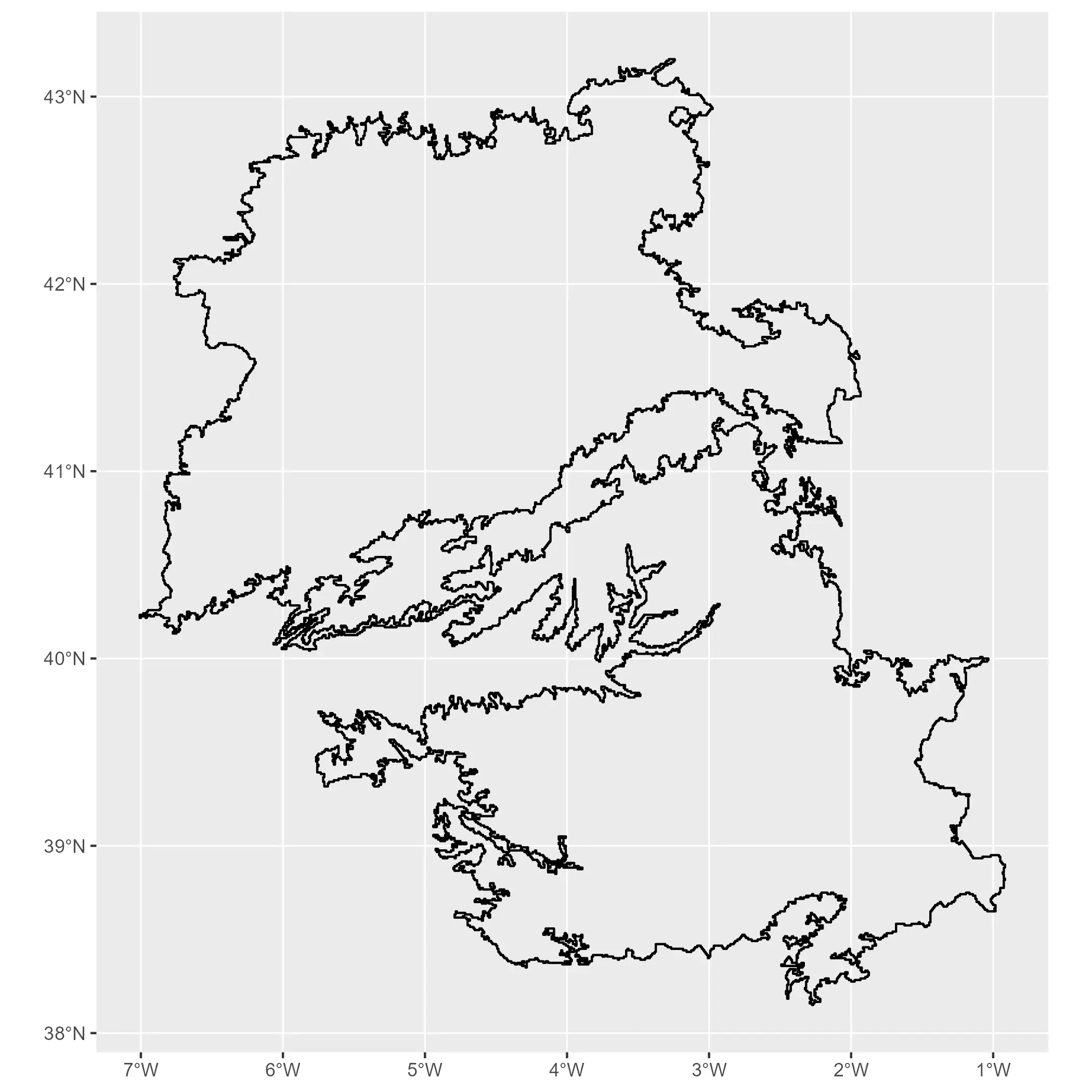We can create a now plot similar to the one produced in the previous post to identify the plain. In first place I create a base layer with a representation of the hillshade, that we would reuse later:

``````

slope <- terrain(r, "slope", unit = "radians")
aspect <- terrain(r, "aspect", unit = "radians")
hill <- shade(slope, aspect, 30, 45)

# normalize names

pal_greys <- hcl.colors(1000, "Grays")

# Index of color by cell
index <- hill %>%
mutate(index_col = rescale(shades, to = c(1, length(pal_greys)))) %>%
mutate(index_col = round(index_col)) %>%
pull(index_col)

# Get cols
vector_cols <- pal_greys[index]

# Need to avoid resampling
# and dont use aes

# Base hill plot
hill_plot <- ggplot() +
geom_spatraster(
data = hill, fill = vector_cols, maxcell = Inf,
alpha = 1
)

hill_plot
``````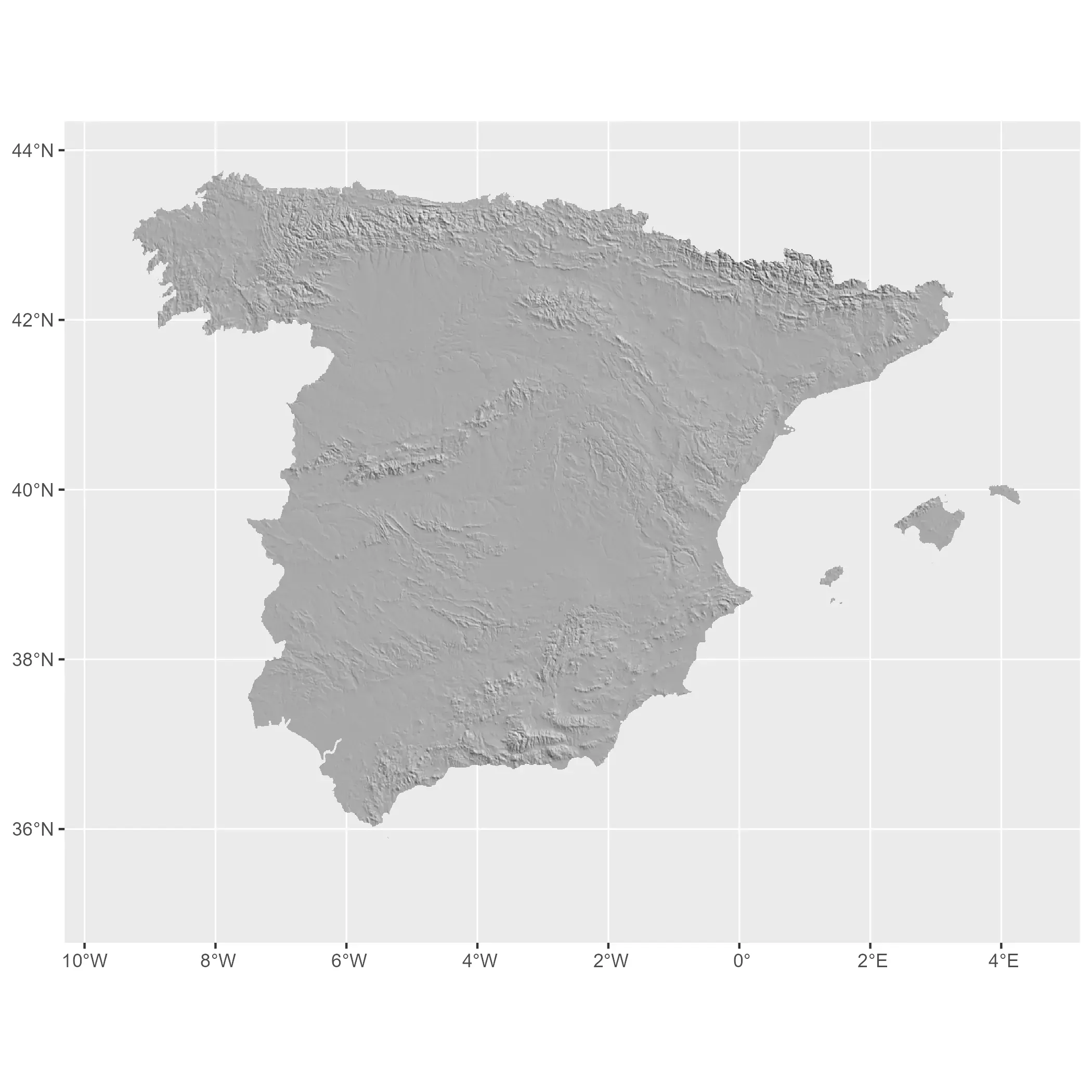And finally we overlay the altitude and the outline of the plain in Spain.

``````# Overlaying and theming

# Aware of limits of the raster

alt_limits <- minmax(r) %>% as.vector()
# Round to lower and higher 500 integer with a min of 0
alt_limits <- pmax(
c(floor(alt_limits / 500), ceiling(alt_limits / 500)) * 500,
0
)

alt_limits
#>     0 3500

base_text_size <- 9

plot_esp <- hill_plot +
geom_spatraster(data = r, maxcell = Inf) +
# Overlay the_plain
geom_spatvector(
data = r_plain,
color = alpha("black", 0.7),
linewidth = 0.15
) +
scale_fill_hypso_tint_c(
palette = "wiki-schwarzwald-cont",
limits = alt_limits,
alpha = 0.4,
breaks = seq(0, 3500, 250),
labels = label_comma()
) +
guides(fill = guide_legend(
title = "   m.",
title.position = "top",
keywidth = .5,
reverse = TRUE,
override.aes = list(alpha = 0.8)
)) +
labs(
title = "Elevation of Spain",
subtitle = "The plain represented with black line"
) +
theme_minimal(base_family = "serif") +
theme(
plot.background = element_rect(fill = "white", color = "white"),
plot.title = element_text(
face = "bold", size = base_text_size * 1.5,
hjust = 0.5
),
plot.subtitle = element_text(
size = base_text_size * 0.9,
hjust = 0.5
),
plot.caption = element_text(
margin = margin(t = base_text_size * 3),
face = "italic"
),
legend.key = element_rect("grey50"),
legend.text = element_text(hjust = 0),
legend.position = "left"
)

plot_esp
``````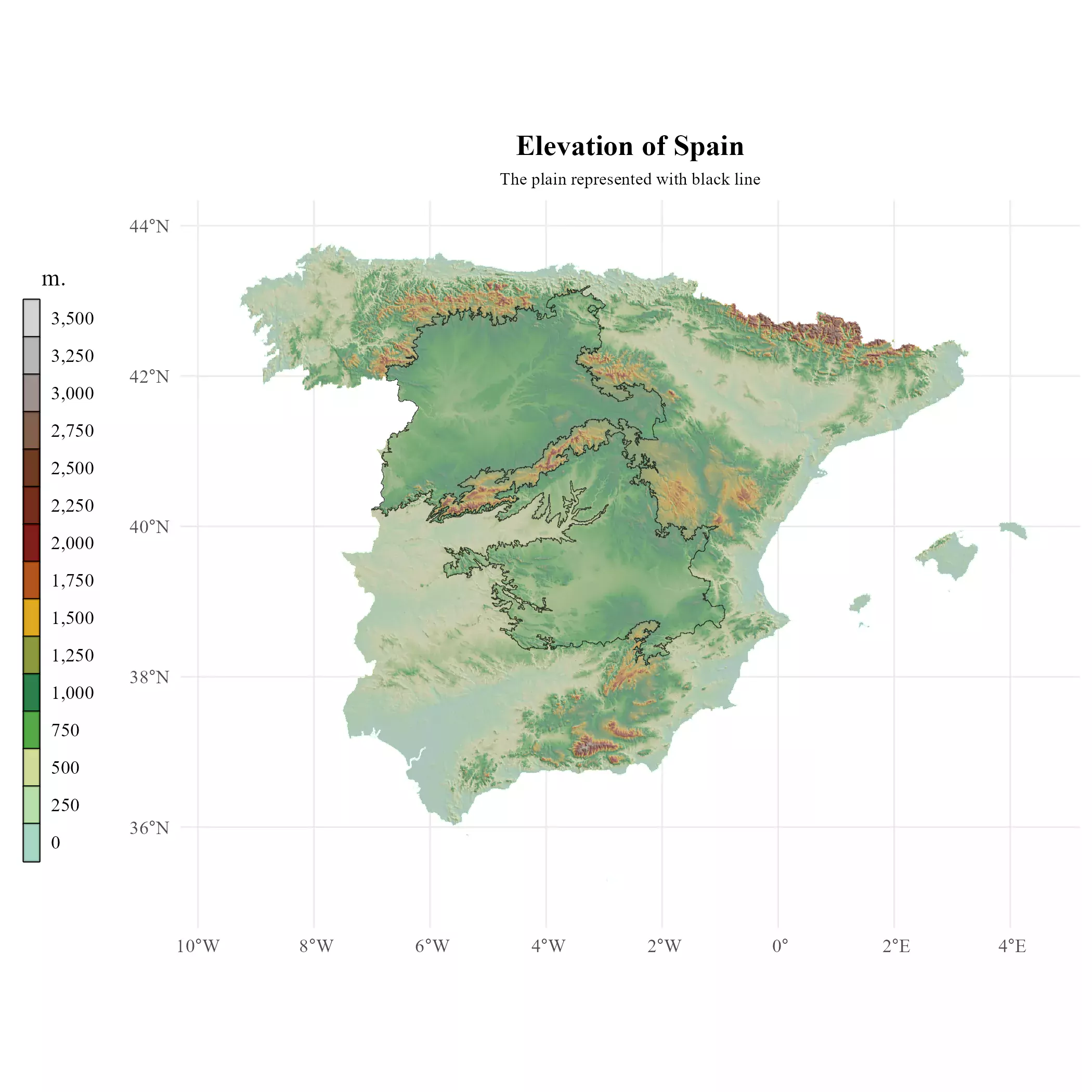## The rain in Spain

Let’s check now wheter the rain falls mainly in the plain or not. We use here `geodata::worldclim_country()` to get the average precipitation by month from WordClim:

``````# Precipitation of Spain

# Get precip data
precip <- geodata::worldclim_country("ESP", "prec", mydir)

precip
#> class       : SpatRaster
#> dimensions  : 1980, 2760, 12  (nrow, ncol, nlyr)
#> resolution  : 0.008333333, 0.008333333  (x, y)
#> extent      : -18.5, 4.5, 27.5, 44  (xmin, xmax, ymin, ymax)
#> coord. ref. : lon/lat WGS 84 (EPSG:4326)
#> source      : ESP_wc2.1_30s_prec.tif
#> names       : ESP_w~rec_1, ESP_w~rec_2, ESP_w~rec_3, ESP_w~rec_4, ESP_w~rec_5, ESP_w~rec_6, ...
#> min values  :           0,           1,           1,           0,           0,           0, ...
#> max values  :         296,         255,         199,         166,         181,         140, ...
``````

We have now a SpatRaster with 12 layers representing the value of each month. So we now just add the values by cell to get the annual average. Note that we also need to normalize the SpatRaster to the projection, extent and resolution of our `hill` object:

``````# Sum all layers
precip_avg <- sum(precip)

precip_avg
#> class       : SpatRaster
#> dimensions  : 1980, 2760, 1  (nrow, ncol, nlyr)
#> resolution  : 0.008333333, 0.008333333  (x, y)
#> extent      : -18.5, 4.5, 27.5, 44  (xmin, xmax, ymin, ymax)
#> coord. ref. : lon/lat WGS 84 (EPSG:4326)
#> source(s)   : memory
#> name        :  sum
#> min value   :    8
#> max value   : 2055

compare_spatrasters(precip_avg, hill)

# Align raster using hill

project(hill) %>%
crop(hill) %>%

# Normalize

``````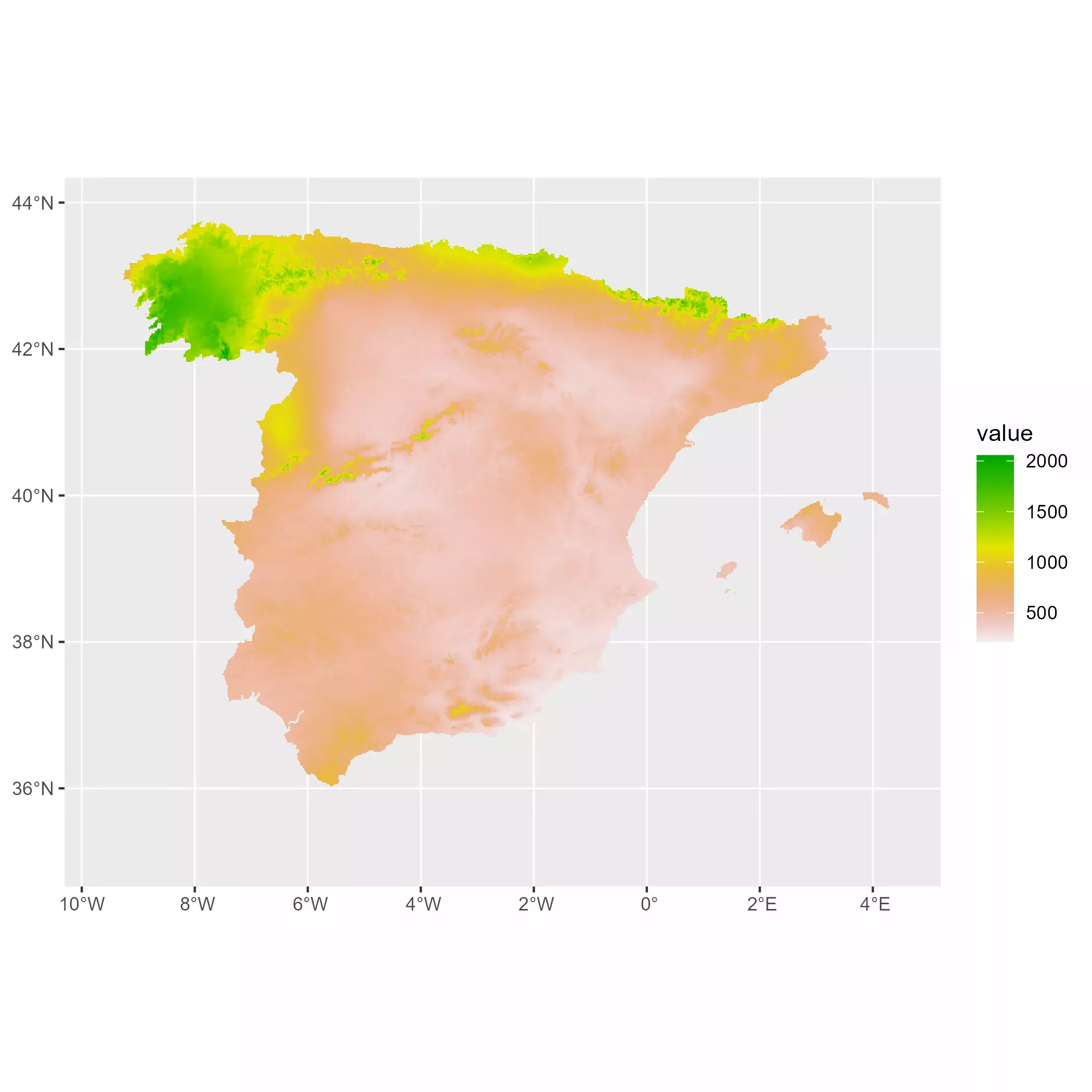### Creating a modified palette

We can now start representing our precipitation map. I chose here to create a custom palette with `colorspace` to better highlight the differences:

``````
mypal <- sequential_hcl(
n = 16,
h = c(320, 80),
c = c(60, 65, 20),
l = c(30, 95), power = c(0.7, 1.3),
rev = TRUE
)

show_col(mypal)
``````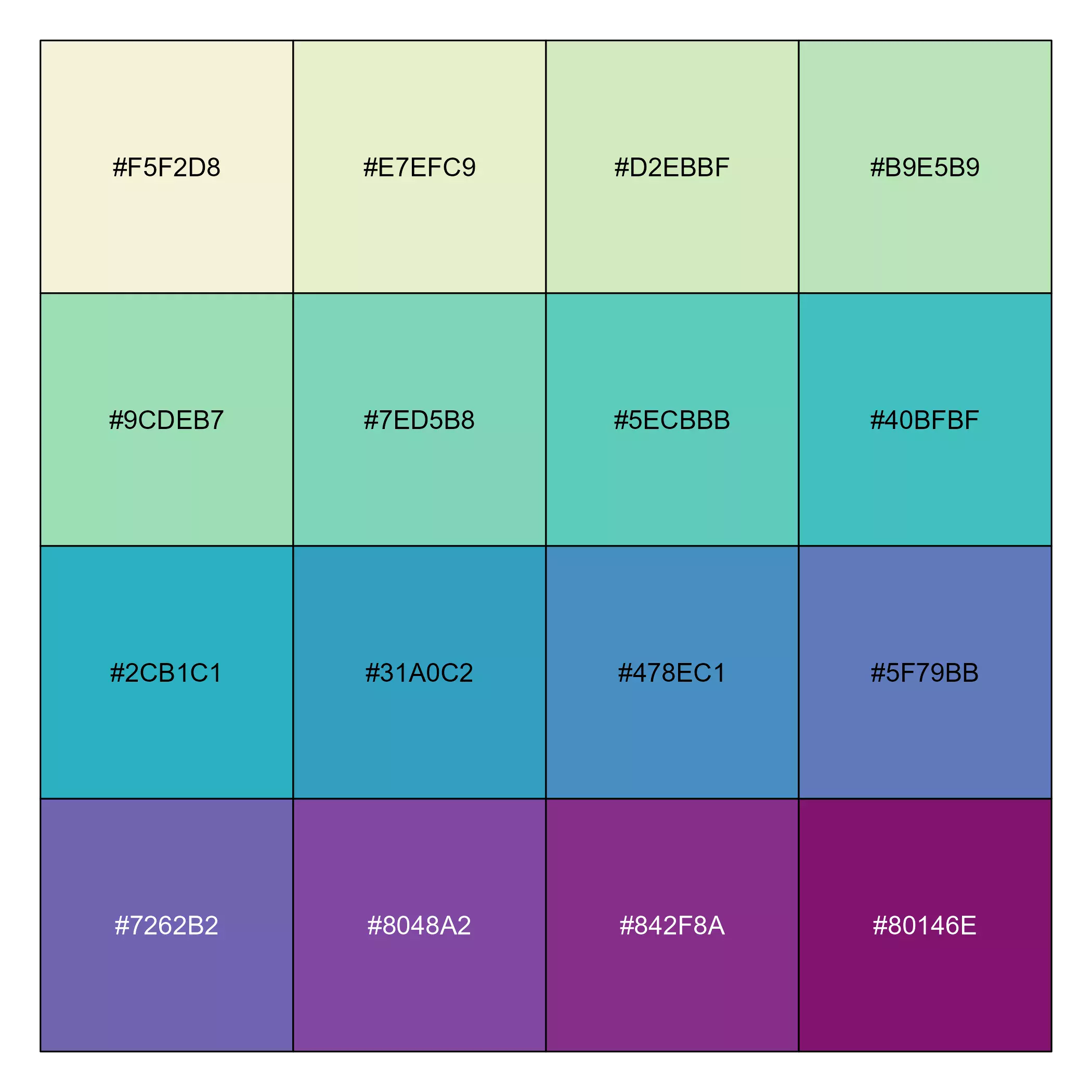And now we can create the final map showing if the rain in Spain stays mainly in the plain:

``````# Precipitation limits, rounded to 100
prec_limits <- floor(as.vector(minmax(precip_avg_mask)) / 100) * 100 + c(0, 100)

meteo_plot <- hill_plot +
geom_spatraster(data = precip_avg_mask, maxcell = Inf) +
# Overlay the_plain
geom_spatvector(
data = r_plain, color = alpha("black", 0.7),
linewidth = .1
) +
# This part is theming only
colours = alpha(mypal, 0.7),
na.value = NA,
labels = label_comma(),
breaks = seq(0, prec_limits, 250)
) +
guides(fill = guide_legend(
direction = "horizontal",
keyheight = .5,
keywidth = 2,
title.position = "right",
label.position = "bottom",
nrow = 1,
family = "serif",
title = " mm.",
override.aes = list(alpha = 0.9)
)) +
labs(
title = "Average yearly precipitation of Spain",
subtitle = "The rain in Spain does not stay mainly in the plain"
) +
theme_minimal(base_family = "serif") +
theme(
plot.background = element_rect(fill = "white", color = "white"),
plot.title = element_text(
face = "bold", size = base_text_size * 1.5,
hjust = 0.5
),
plot.subtitle = element_text(
size = base_text_size,
hjust = 0.5
),
axis.text = element_text(size = base_text_size * 0.7, face = "italic"),
legend.key = element_rect("grey50"),
legend.position = "bottom",
legend.title = element_text(size = base_text_size * .7),
legend.text = element_text(size = base_text_size * .7),
legend.spacing.x = unit(0, "pt")
)

meteo_plot
``````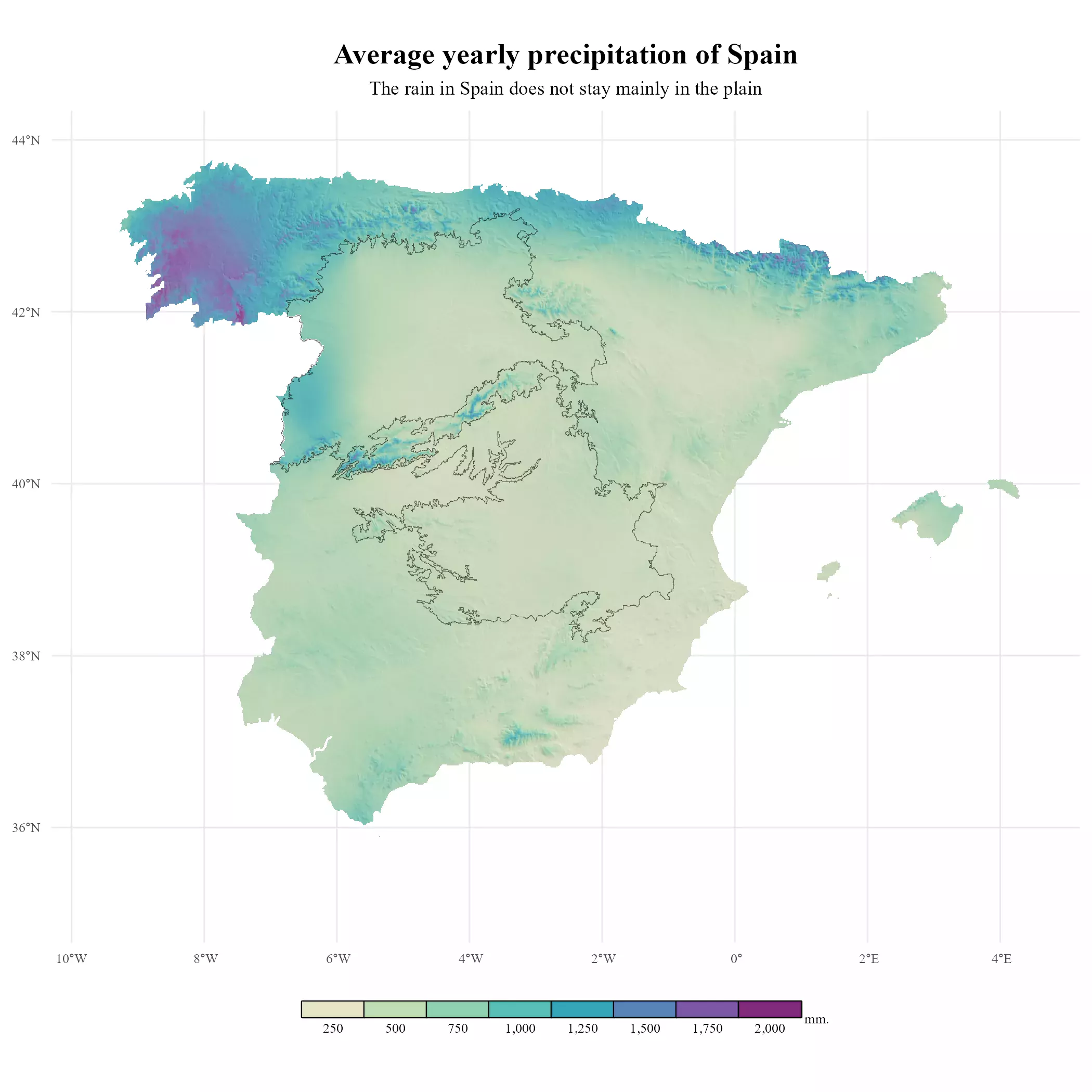We can now check that the rain in Spain falls mainly in the Atlantic coast (North of Spain) and specifically in Galicia. That’s why in Spanish the lyrics The rain in Spain stays mainly in the plain were translated into:

La lluvia en Sevilla es una pura maravilla.

That can be translated as “The rain in Seville is a true marvel”. And it is, indeed. Seville (located in the south on the Guadalquivir Valley has circa 50 rainy days per year, featuring very hot and dry summers.

## Marginal plots (finally)

We can now start profiling our final plot. The idea is to create two bar charts, representing the value to be plotted (in this case, average annual precipitation) by longitude and latitude.

But first we add some additional margins and title axes to the main plot, so we can insert those marginal plots easily on our main plot:

``````# Now we can add titles on the secondary axis

plot_main <- meteo_plot +
xlab("") +
ylab("") +
# titles on secondary axis, for later
scale_x_continuous(sec.axis = dup_axis(
name = "avg. precipitation by longitude"
)) +
scale_y_continuous(sec.axis = dup_axis(
name = "avg. precipitation by latitude"
)) +
theme(
axis.title.x = element_text(
margin = margin(t = base_text_size),
size = base_text_size * 0.9, face = "italic"
),
axis.title.y = element_text(
angle = 270,
margin = margin(
l = base_text_size,
t = base_text_size
),
size = base_text_size * 0.9, face = "italic"
)
)

plot_main
``````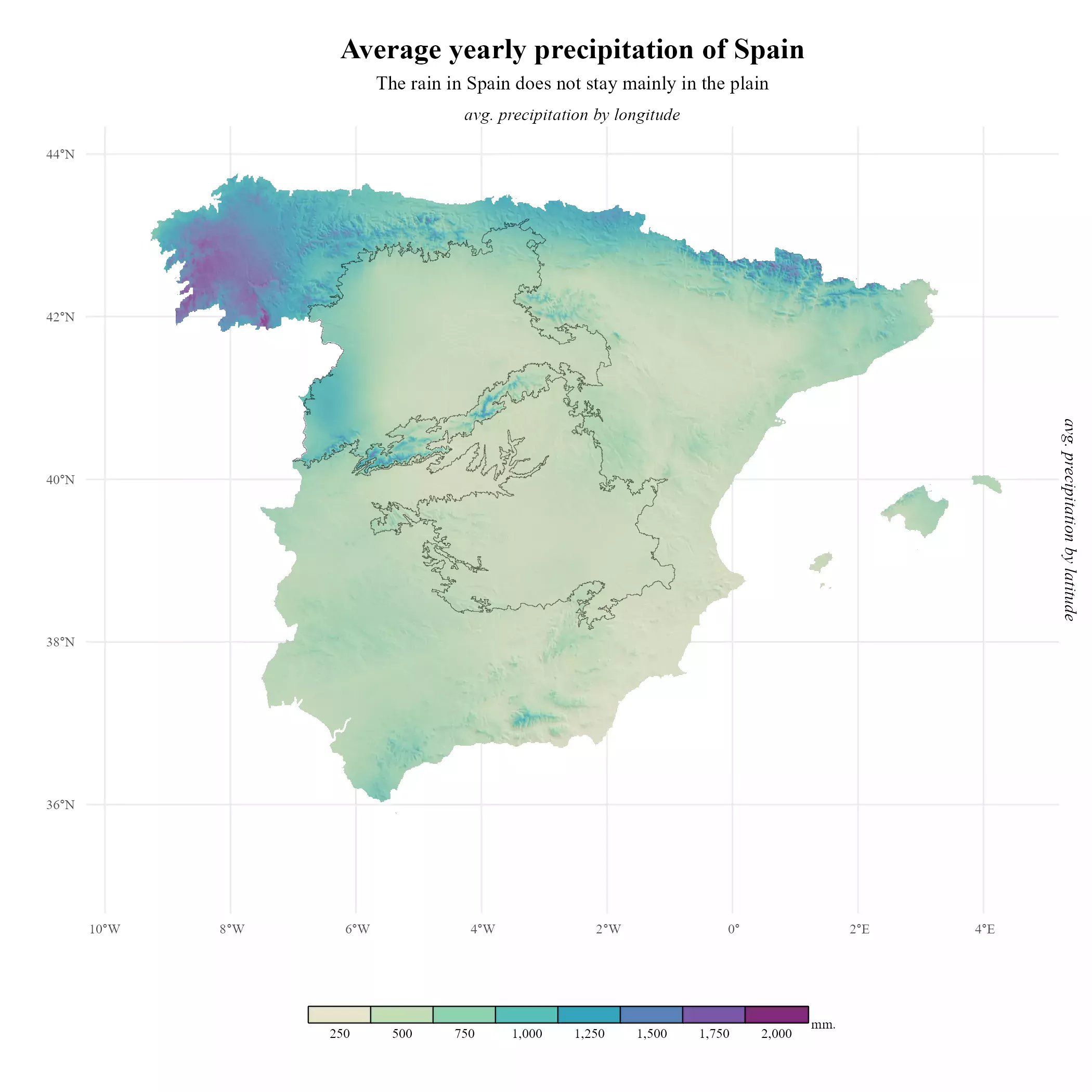### Profiling marginal plots

On the following code, I am just drafting how the marginal plots would look like, so we can have a preview of the final result:

``````
# Profiling marginal plots

# Getting averages by x,y
as_tibble(xy = TRUE) %>%
drop_na() %>%
group_by(x) %>%
summarise(avg = mean(prec))

as_tibble(xy = TRUE) %>%
drop_na() %>%
group_by(y) %>%
summarise(avg = mean(prec))

# Cowplot would delete axis, we create an axis at 1000 and 2000
br_4marginal <- c(1000, 2000)

labs <- data.frame(labs = paste(
prettyNum(br_4marginal, big.mark = " "),
"mm."
))

labs\$for_x <- max(marg_x\$x) - diff(range(marg_x\$x)) * 0.05
labs\$for_y <- min(marg_y\$y) + diff(range(marg_y\$y)) * 0.05
labs\$y <- br_4marginal

# Profiling
ggplot() +
geom_col(
data = marg_x,
aes(x, avg, fill = avg),
color = NA,
show.legend = FALSE
) +
geom_text(
data = labs, aes(x = for_x, y = y, label = labs),
nudge_y = 100,
size = 3
) +
colours = alpha(mypal, 0.9),
na.value = NA,
labels = label_comma(),
limits = prec_limits
) +
scale_y_continuous(
breaks = br_4marginal,
limits = c(0, max(br_4marginal) * 1.5)
) +
theme_void() +
theme(panel.grid.major.y = element_line(
colour = "grey50",
linetype = "dashed"
))
``````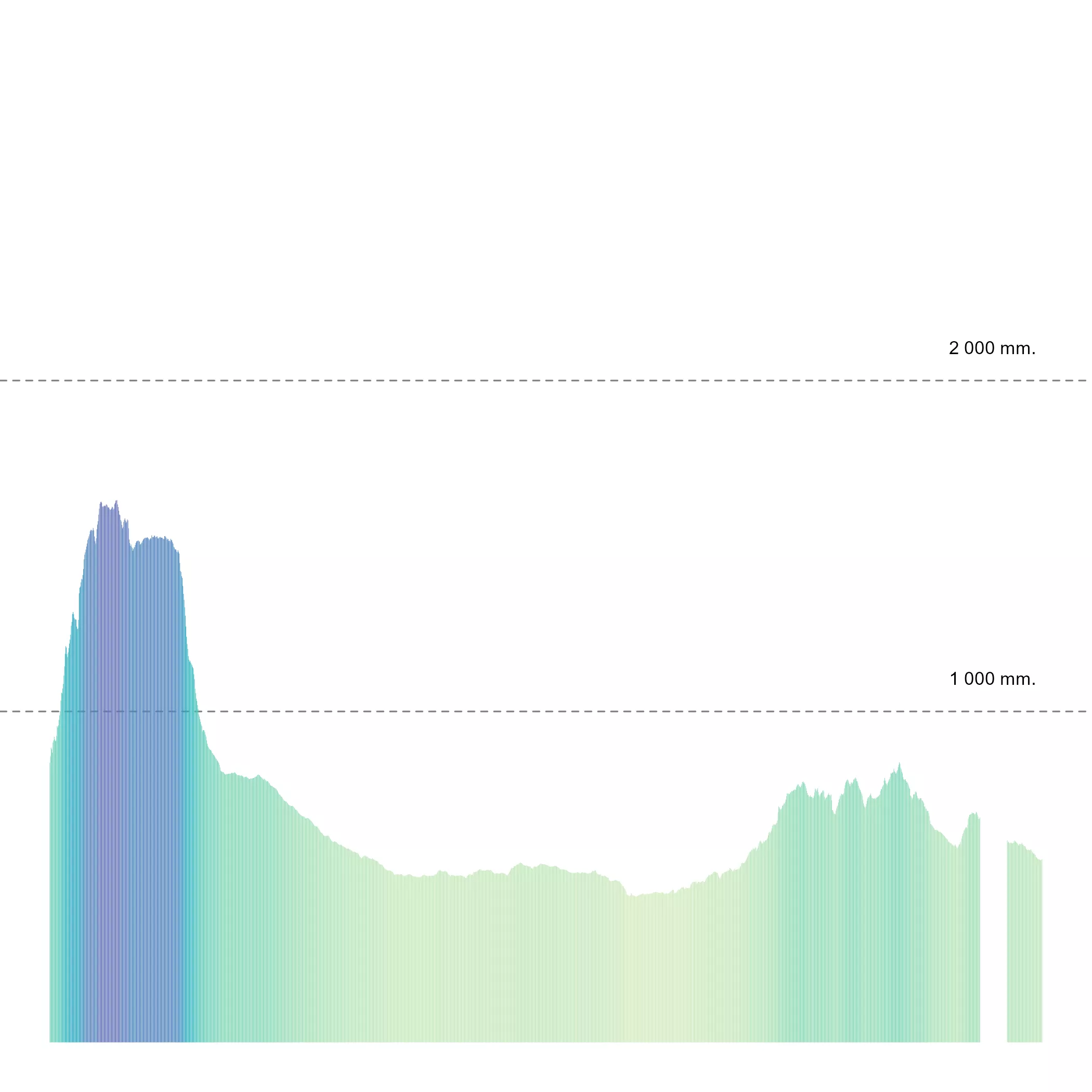``````
ggplot() +
geom_col(
data = marg_y,
aes(y, avg, fill = avg),
color = NA,
show.legend = FALSE
) +
geom_text(
data = labs, aes(x = for_y, y = y, label = labs),
nudge_y = 100,
angle = 270,
size = 3
) +
colours = alpha(mypal, 0.9),
na.value = NA,
labels = label_comma(),
limits = prec_limits
) +
scale_y_continuous(
breaks = br_4marginal,
limits = c(0, max(br_4marginal) * 1.2)
) +
coord_flip() +
theme_void() +
theme(panel.grid.major.x = element_line(
colour = "grey50",
linetype = "dashed"
))
``````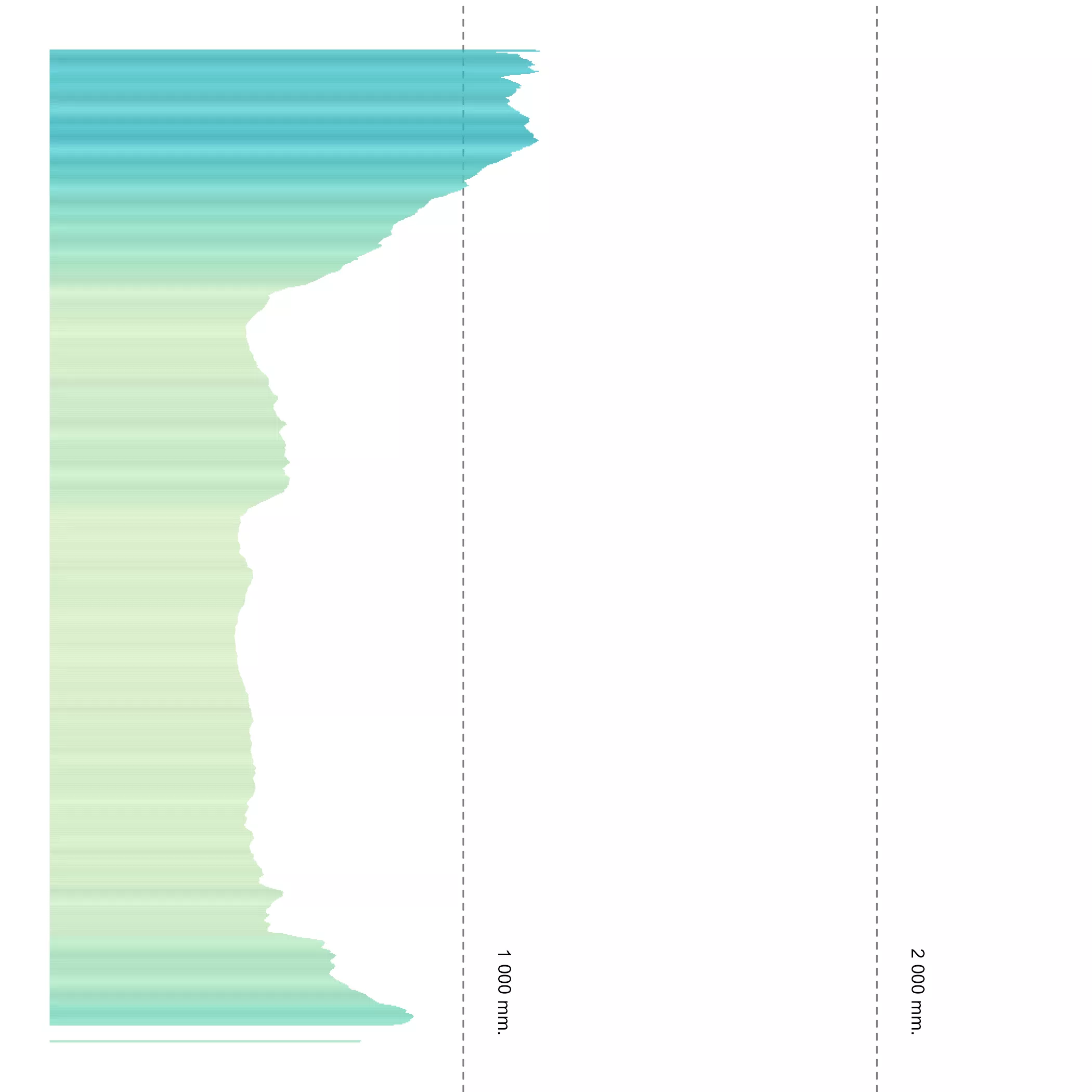### Putting all the pieces together

Finally, we would use `cowplot::axis_canvas()` to create the marginal plots as we want:

``````
# Last step: We combine plots

# Marginal plots
plot_x <- axis_canvas(plot_main, axis = "x") +
geom_col(
data = marg_x,
aes(x, avg, fill = avg),
color = NA,
show.legend = FALSE
) +
geom_text(
data = labs, aes(x = for_x, y = y, label = labs),
# Adjust the position of the labels
nudge_y = 300,
family = "serif",
fontface = "italic",
size = base_text_size * 0.2
) +
colours = alpha(mypal, 0.9),
na.value = NA,
labels = label_comma(),
limits = prec_limits
) +
scale_y_continuous(
breaks = br_4marginal,
limits = c(0, max(br_4marginal) * 1.5)
) +
theme_void() +
theme(panel.grid.major.y = element_line(
colour = "grey50",
linetype = "dashed",
linewidth = 0.1
))

plot_x
``````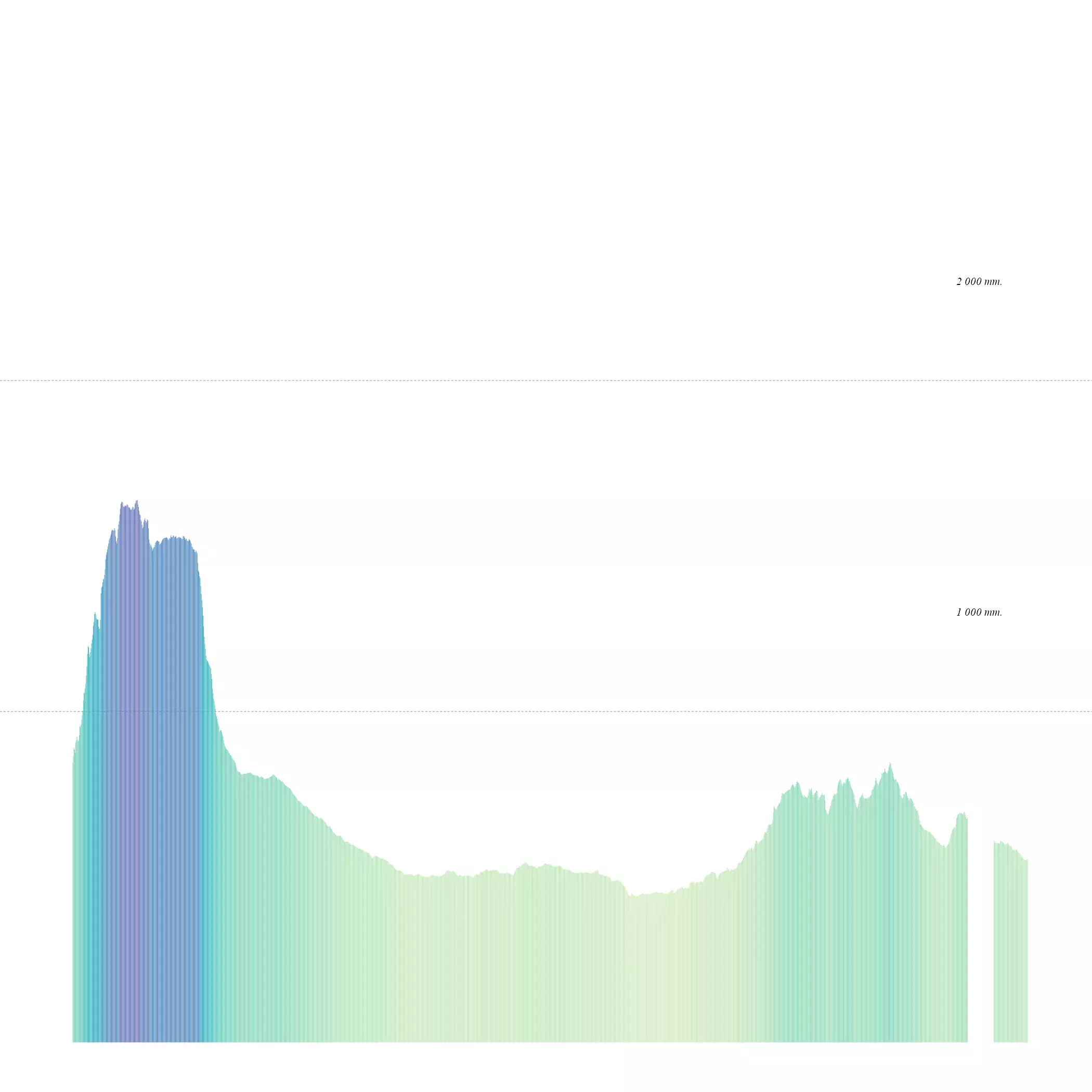``````
plot_y <- axis_canvas(plot_main, axis = "y", coord_flip = TRUE) +
geom_col(
data = marg_y,
aes(y, avg, fill = avg),
color = NA,
show.legend = FALSE
) +
geom_text(
data = labs, aes(x = for_y, y = y, label = labs),
# Adjust the position of the labels
nudge_y = 300,
angle = 270,
family = "serif",
fontface = "italic",
size = base_text_size * 0.2
) +
limits = prec_limits,
colours = alpha(mypal, 0.9),
na.value = NA,
labels = label_comma()
) +
scale_y_continuous(
breaks = br_4marginal,
limits = c(0, max(br_4marginal) * 1.5)
) +
coord_flip() +
theme_void() +
theme(panel.grid.major.x = element_line(
colour = "grey50",
linetype = "dashed",
linewidth = 0.1
))
plot_y
``````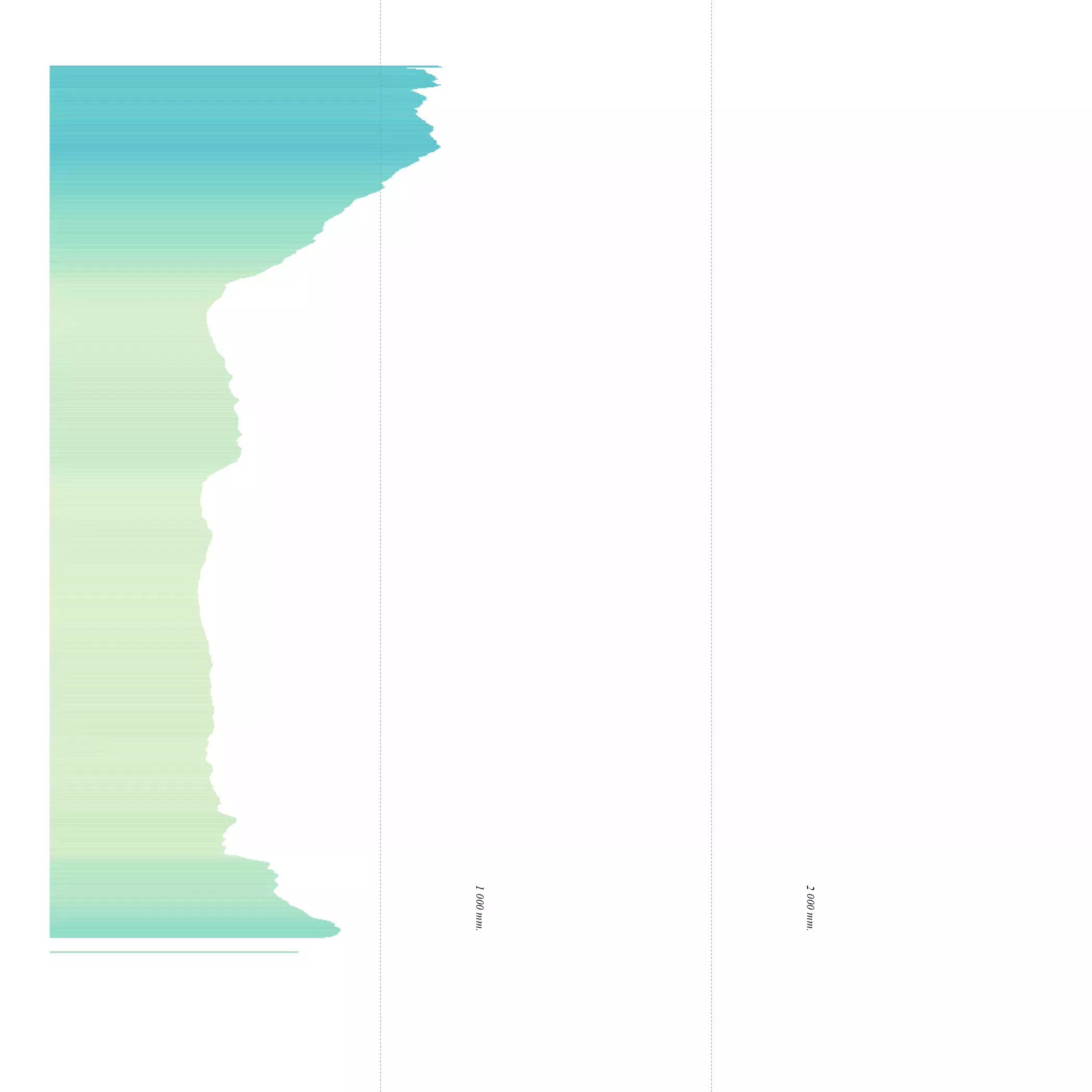And insert everything in the main plot. See the final result:

``````
# Combine all plots into one
sizes_axis <- grid::unit(.3, "null")

plot_final <- insert_xaxis_grob(plot_main, plot_x,
position = "top",
height = sizes_axis
)
plot_final <- insert_yaxis_grob(plot_final, plot_y,
position = "right",
width = sizes_axis * 1.25
)

gg_final <- ggdraw(plot_final)
gg_final
``````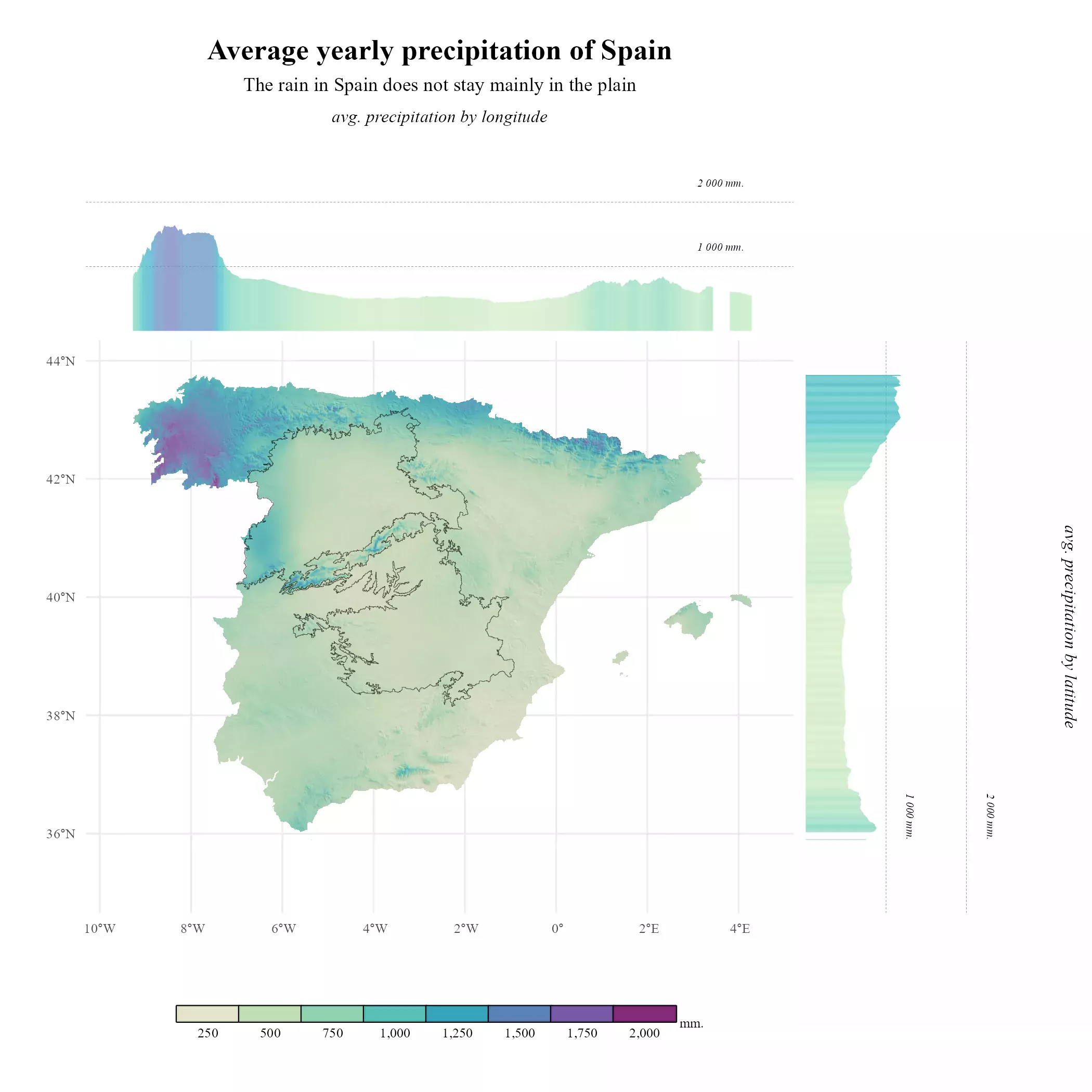And with a bit of effort we got it.

## Recap

Much of the code we have created relates with the theming and labels of the plot. Here you can find a simplified version:

Simplified version
``````
# Libraries
# Data manipulation
library(terra)
library(tidyterra)
library(dplyr)

# Get the data
library(geodata)

# Plotting
library(ggplot2)
library(scales)
library(cowplot)
library(colorspace)

# Get the data
mydir <- "~/R/mapslib/misc"

r <- elevation_30s("ESP", path = mydir) %>%
rename(alt = 1) %>%
mutate(alt = pmax(0, alt))

slope <- terrain(r, "slope", unit = "radians")
aspect <- terrain(r, "aspect", unit = "radians")
hill <- shade(slope, aspect, 30, 45)

# normalize names

pal_greys <- hcl.colors(1000, "Grays")

# Index of color by cell
index <- hill %>%
mutate(index_col = rescale(shades, to = c(1, length(pal_greys)))) %>%
mutate(index_col = round(index_col)) %>%
pull(index_col)

# Get cols
vector_cols <- pal_greys[index]

# Base hill plot
hill_plot <- ggplot() +
geom_spatraster(
data = hill, fill = vector_cols, maxcell = Inf,
alpha = 1
) +
theme_minimal()

# Overlay
precip <- geodata::worldclim_country("ESP", "prec", mydir)
precip_end <- sum(precip) %>%
project(hill) %>%
crop(hill) %>%
rename(prec = 1)

p_range <- as.vector(minmax(precip_end))

mypal <- sequential_hcl(
n = 16,
h = c(320, 80),
c = c(60, 65, 20),
l = c(30, 95), power = c(0.7, 1.3),
rev = TRUE
)

base_plot <- hill_plot +
geom_spatraster(data = precip_end, maxcell = Inf) +
colors = alpha(mypal, 0.7), na.value = NA,
limits = p_range
)

# Marginal plots
# Data
marg_x <- precip_end %>%
as_tibble(xy = TRUE) %>%
drop_na() %>%
group_by(x) %>%
summarise(avg = mean(prec))

marg_y <- precip_end %>%
as_tibble(xy = TRUE) %>%
drop_na() %>%
group_by(y) %>%
summarise(avg = mean(prec))

plot_x <- axis_canvas(base_plot, axis = "x") +
geom_col(
data = marg_x,
aes(x, avg, fill = avg),
color = NA,
show.legend = FALSE
) +
colours = alpha(mypal, 0.9),
na.value = NA,
limits = p_range
) +
theme_void()

plot_y <- axis_canvas(base_plot, axis = "y", coord_flip = TRUE) +
geom_col(
data = marg_y,
aes(y, avg, fill = avg),
color = NA,
show.legend = FALSE
) +
colours = alpha(mypal, 0.9),
na.value = NA,
limits = p_range
) +
theme_void() +
coord_flip()

# All pieces together
sizes_axis <- grid::unit(.3, "null")

plot_final_simp <- insert_xaxis_grob(base_plot, plot_x,
position = "top",
height = sizes_axis
)
plot_final_simp <- insert_yaxis_grob(plot_final_simp, plot_y,
position = "right",
width = sizes_axis * 1.25
)

gg_final_simp <- ggdraw(plot_final_simp)
gg_final_simp
``````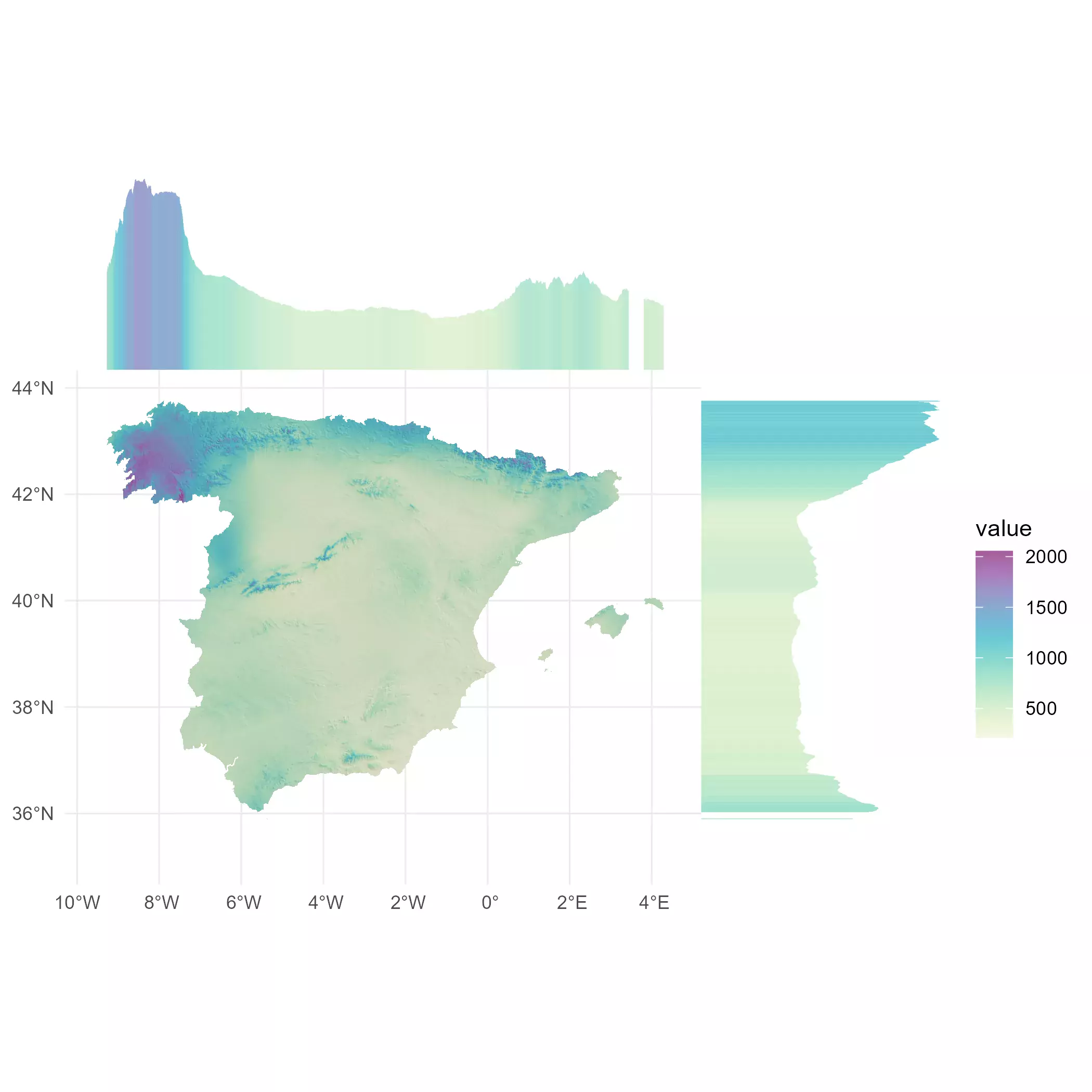Related